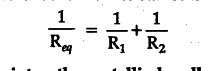# What is difference in temperature total rate of heat transfer and equivalent thermal resistance when two conductors are placed on the side of each other with ends at the same reservoirs of heat?

When two conductors are placed on the side of each other with their ends at the same reservoirs of heat then,
(i) Difference in temperature is same.
(ii) The total rate of heat transfer is the sum of the heat transfer in them.
(iii) The equivalent thermal resistance is found from